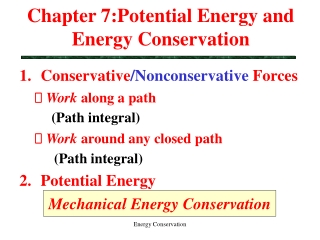DownloadDownload PresentationChapter 7:Potential Energy and Energy Conservation

# Chapter 7:Potential Energy and Energy Conservation

Download Presentation## Chapter 7:Potential Energy and Energy Conservation

- - - - - - - - - - - - - - - - - - - - - - - - - - - E N D - - - - - - - - - - - - - - - - - - - - - - - - - - -
##### Presentation Transcript

1. Chapter 7:Potential Energy and Energy Conservation • Conservative/Nonconservative Forces  Work along a path (Path integral)  Work around any closed path (Path integral) • Potential Energy Mechanical Energy Conservation Energy Conservation

2. y Work Done bythe Gravitational Force (I) Near the Earth’s surface l (Path integral) Energy Conservation

3. y Work Done bythe Gravitational Force (II) Near the Earth’s surface (Path integral) dl Energy Conservation

4. Wg < 0 if y2 > y1 Wg > 0 if y2 < y1 The work done by the gravitational force depends only on the initial and final positions.. Work Done bythe Gravitational Force (III) Energy Conservation

5. Wg(ABCA) =Wg(AB) + Wg(BC) + Wg(CA) =mg(y1 – y2) + 0 + mg(y2- y1) = 0 Work Done bythe Gravitational Force (IV) C B dl A Energy Conservation

6. Energy Conservation

7. Wg = 0 for a closed path The gravitational force is a conservative force. Work Done bythe Gravitational Force (V) Energy Conservation

8. Work Done by Ff (I) (Path integral) - μmg L L depends on the path. LB Path B Path A LA Energy Conservation

9. The work done by the friction force depends on the path length. The friction force: (a) is a non-conservative force; (b) decreases mechanical energy of the system. Work Done by Ff (II) Wf = 0 (any closed path) Energy Conservation

10. Example 1 A 1000-kg roller-coaster car moves from point A, to point B and then to point C. What is its gravitational potential energy at B and C relative to point A? Energy Conservation

11. Wg(ABC) =Wg(AB) + Wg(BC) =mg(yA- yB) + mg(yB - yC) = mg(yA - yC) Wg(AC) = Ug(yA) – Ug(yC) y B A dl B C A Energy Conservation

12. Work-Energy Theorem Conservation ofMechanical Energy (K+U) Wconservative (AC)= UA – UC If Wnet = Wconservative , then KC – KA = Wnet (AC)= UA – UC KC + UC = KA + UA Energy Conservation

13. Energy Conservation

14. Example 1 X h/2 U K X Energy Conservation

15. Work Done by FS using Uel(x) y x WS = Uel(xi ) – Uel(xf ) where Uel(x) = (1/2) k x2 Energy Conservation

16. Glossary • K: Energy associated with the motion of an object. • U: Energy stored in a system of objects • Can either do work or be converted to K. • Q: Thermal Energy (Internal Energy)  The energy of atoms and molecules that make up a body. Energy Conservation

17. Work Done by Fg using Ug(h) Wg = U(hi ) – U(hf ) where: Ug(h) = m g h (near the Earth’s surface) h1 h3 h4 = 0 h2 Energy Conservation

18. Example 2 A roller coaster sliding without friction along a circular vertical loop (radius R) is to remain on the track at all times. Find the minimum release height h. A C B Energy Conservation

19. Energy Conservation

20. Example 2 (cont’d) A roller coaster sliding without friction along a circular vertical loop (radius R) is to remain on the track at all times. Find the minimum release height h. A v C (2) mv2/R = mg FN = 0 mg (1) UA = UC + KC B Energy Conservation

21. Energy Conservation

22. Example 4 Circular Motion & WT = 0 A C vC = ? B vB = ? Energy Conservation

23. Energy Conservation

24. Energy Conservation

25. Energy Conservation

26. Work Done by FG using UG(r) WG = UG(ri ) - UG(rf ) where: UG(r)= - GmME / r (dl)r = (dl)f Energy Conservation

27. Work Done by FS using Uel(x) y x WS = Uel(xi ) – Uel(xf ) where Uel(x) = (1/2) k x2 Energy Conservation

28. (4) W-E Theorem to find v2(= 1.93 m/s). (1) F.B.D. (2) W by each force (3) Wnet Example 2 v2 = ? FN FP v1 = 0 motion d=5m Ff Fg mk= 0.100 Wg = Ug2 – Ug1 or Wg = m g d (FP)x = FP /cosq Energy Conservation

29. Example 3 mk = ? vf = 0 d Energy Conservation

30. Example 5 How much work must the satellite’s engines perform to move its satellite (mass m = 300 kg) from a circular orbit of radius rA = 8000 km about the Earth to another circular orbit of radius rC = 3 rA? vA = ? vC = ? Energy Conservation

31. y Gravitational Potential Energy Near the Earth’s surface dl Energy Conservation